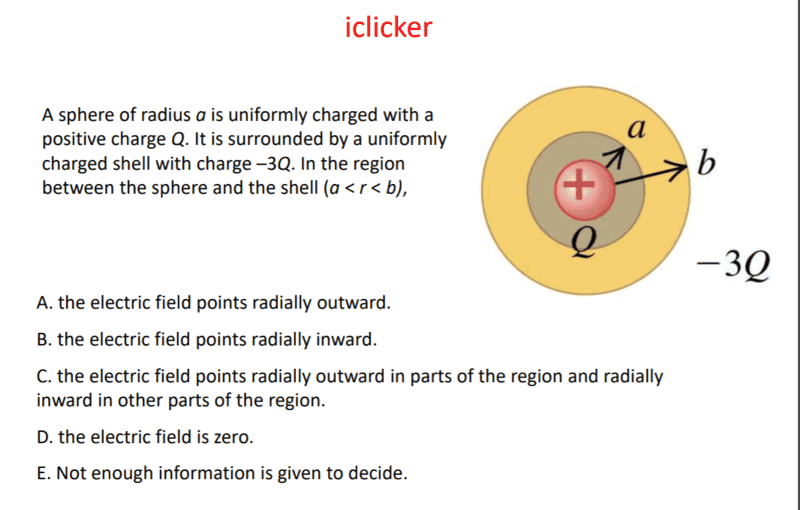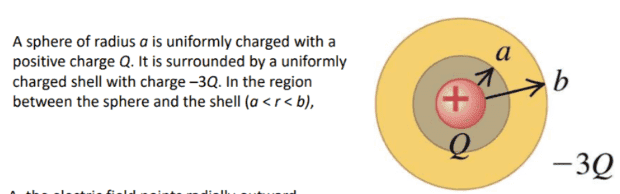# Electric Field Inside Concentric Spheres

## Homework Statement## The Attempt at a Solution

I am having trouble figuring out why the answer is A) the electric field points radially between A and B. I think it is because since the point between A and B is mostly negative, the electric field would point outwords more strongly than it would for the positive charges which point the otherway. Is that why the electric field would point outward between A and B?

#### Attachments

Homework Helper
Gold Member
This problem is most readily computed using Gauss' law. That is by far the easiest and clearest way of showing the answer. Have you seen Gauss' law yet in your coursework? ## \\ ## Edit: I read this more carefully=after removing a couple of statements I made that are inapplicable= Gauss' law does give the answer. Without Gauss' law, it would be difficult to compute it.

Last edited:
This problem is most readily computed using Gauss' law. That is by far the easiest and clearest way of showing the answer. Have you seen Gauss' law yet in your coursework? ## \\ ## Edit: I read this more carefully=after removing a couple of statements I made that are inapplicable= Gauss' law does give the answer. Without Gauss' law, it would be difficult to compute it.

Yes, i learned about Gauss's law. Then i would have to find the charge based on the charge density? Wouldn't there be two regions of differing charge density?

edit : E = (p*V1 + p*V2)/ ((4pi*r^2)*episilon*)

gneill
Mentor
Something is bothering me regarding the figure accompanying the problem statement.The text states that there is a charged sphere surrounded by a charged shell, and refers to the region between them. To me is seems that the pinkish-red object at the center would be the inner sphere and the tan region the surrounding shell. The region between would be the darker grey-ish part. But the radius indicators suggest that the inner sphere reaches the inner surface of the shell. Are the radius vectors and charge labels part of the original figure?

#### Attachments

•But the radius indicators suggest that the inner sphere reaches the inner surface of the shell. Are the radius vectors and charge labels part of the original figure?

I guess that is why im confused. The pic was part of a lecture slides since it was a iclicker question. There is no original figure unfortunately. I do know that the answer is A)

Edit: I get it now. I don't think the picture is really helpful. Redrawing the diagram based on the description, it makes more sense that the efield points outward.

Homework Helper
Gold Member
If you draw a spherical Gaussian shell of radius ## a<r<b ##, there will be radii where the negative charge enclosed is minimal, e.g. radii ## r \approx a ##, but also radii where the negative charge enclosed exceeds the positive charge ## +Q ## at the center, e.g. for ## r \approx b ##. That should help determine the answer. If you know how to work Gauss' law proficiently, the answer should be obvious. ## \\ ## One question I also have, as did @gneill above: They misstated the problem: Are they interested in the region between the sphere and the outer shell, or in the outer shell region of ## a<r<b ##? The answer is different for these two regions.

Last edited:
gneill
Mentor
Another approach to the problem is to consider that inside a spherical shell of charge the field due to that shell
One question I also have, as did @gneill above: They misstated the problem: Are they interested in the region between the sphere and the shell, or in the outer shell region of a<r<ba<r<b a
If the "known" answer is ##A##, then we can decide between those choicesIf the "known" answer is ##A##, then we can decide between those choices•